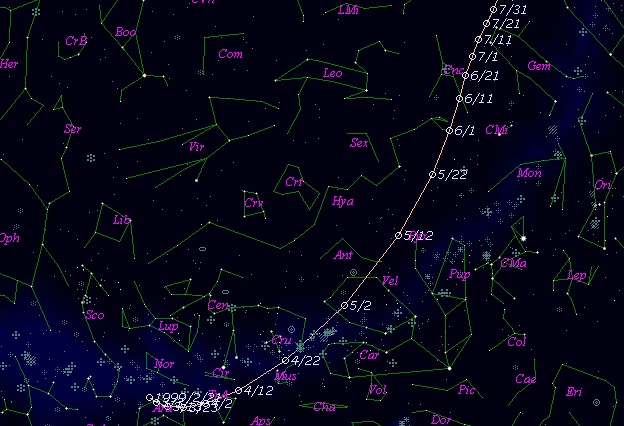# C/1999 H1 ( Lee )###Profile

 Discovery Date April 16, 1999 Magnitude 9 mag Discoverer Steven Lee (Mudgee, N.S.W.)

###Pictures###Orbital Elements

```   The following improved orbital elements by Kenji Muraoka, are from
888 observations 1999 Apr. 16 to Dec. 10, including 8 Planets, Moon
and 3 minor planets perturbations and non-gravitational effect of
style II.  The mean residual is +/- 0.78 arc seconds.

Epoch  =  1999 July  1.0  TT       JDT = 2451360.5
T  =  1999 July 11.17316       +/- 0.00019 (m.e.) TT
Peri. =   40.70436                +/- 0.00060
Node  =  162.65140                +/- 0.00018   (2000.0)
Incl. =  149.35276                +/- 0.00009
q  =    0.7080729              +/- 0.0000070 AU
e  =    0.9996221              +/- 0.0000050
1/a  =   +0.0005337              +/- 0.0000071 1/AU
A1  =   +2.65                   +/- 0.28
A2  =   -0.1028                 +/- 0.0527
( P  =  81103 years )
```

###Finding Charts###Magnitudes Graph

```        m1 = 6.3 + 5 log d + 14.0 log r  [   ,-35]  (             - 1999 June  6)
m1 = 6.3 + 5 log d +  5.0 log r  [-35, 40]  (1999 June  6 - 1999 Aug. 20)
m1 = 6.0 + 5 log d + 14.0 log r  [ 40,120]  (1999 Aug. 20 - 1999 Nov.  8)
m1 = 8.9 + 5 log d + 10.0 log r  [120,   ]  (1999 Nov.  8 -             )
```##### The orbital elements are calculated by Kenji Muraoka. The charts are made with StellaNavigator Ver.2.0 for Windows (AstroArts / ASCII). The magnitudes graphs are made with Comet for Windows.